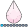## Hazard Ratio Plot

Hello,

I am trying to create a line graph showing Hazard Ratio over time  using the following axis below:

Hazard Ratio    6

5

4

3

2

1

0

0        20       40       60       80       100     120    140

Time

I have create the hazard ratios for the tables but am unsure now how to plot them and what procedure I need to use to create the plot.  If anyone can help to get me started that would be great.

Here is the syntax used to create ratios:

Proc phreg data=&infile;

class exposure;

Model personyrs*outcome(0) = exposure &covariates trtt /RISKLIMITS;;

trtt = exposure*log(PersonTime);

test_proportionality: TEST trtt;

ods output ParameterEstimates=HR&infile;

run;

3 REPLIES 3djrisks
Barite | Level 11

## Re: Hazard Ratio Plot

Hello, I'm guessing you can use the Series statement within SGPLOT to get the plot that you want.

Can you please include the &infile dataset or the HR&infile dataset so that I can verify?

## Re: Hazard Ratio Plot

I am not sure how to attach a file, so have copied and pasted the information from the dataset HR&infile created by the proc phreg procedure

Parameter      Standard                                           Hazard      95% Hazard Ratio

Parameter             DF      Estimate         Error    Chi-Square    Pr > ChiSq       Ratio      Confidence Limits     Label

Exposure           0     1     -12.89787       0.26728     2328.6241        <.0001       0.000       0.000       0.000    Exposure 0

Age_At_Index           1       0.04090       0.00188      473.8845        <.0001       1.042       1.038       1.046

trtt                              1      -2.24461       0.04631     2349.3378        <.0001       0.106       0.097       0.116djrisks
Barite | Level 11

## Re: Hazard Ratio Plot

Okay, the output for the table you have included above is usually for a forest plot. Maybe that is the plot you are after? An example of a forest plot can be found here: http://blogs.sas.com/content/graphicallyspeaking/2013/01/06/forest-plot-with-sas-9-3/

In the figure you want to produce, you have time on the x-axis. Have you also outputted a dataset that includes the time and the hazard ratio's?

Discussion stats
• 3 replies
• 2545 views
• 0 likes
• 2 in conversation